# Subtraction without Regrouping

Subtraction without Regrouping• We will subtract 12 from 35 by partitioning the numbers into their tens and units.
• The number 35 is made up of 5 individual ones and 3 groups of ten.
• The number 12 is made up of 2 individual ones and 1 group of ten.
• We start with the units column and subtract the number below from the number above.
• In the units column, 5 – 2 = 3.
• In the tens column, 3 – 1 = 2.
• There are 2 groups of ten and 3 units remaining.
• 35 – 12 = 23.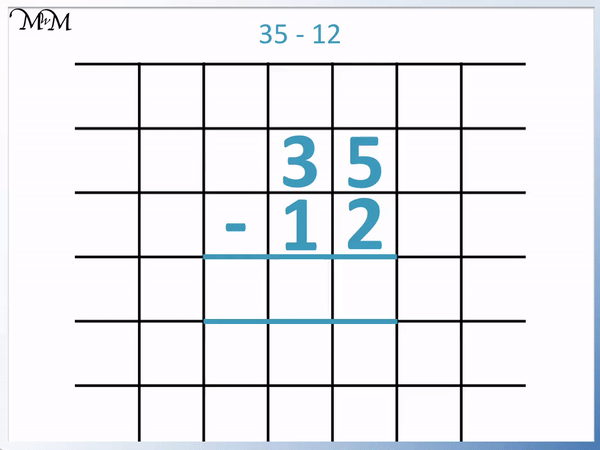• We set up the vertical column subtraction by writing the digits of each number above each other, with one digit per box.
• We write the larger number above the smaller number.
• We subtract the digits below from the digits above, working from right to left.
• 5 – 2 = 3.
• 3 – 1 = 2.

Write the larger number above the smaller number, lining up the digits.

Subtract the digits below from the digits above, working from right to left.#### Vertical Column Subtraction without Regrouping: Interactive Questions

Vertical Column Subtraction without Regrouping: Interactive Question Generator

#### Vertical Column Subtraction Workbook

Download our printable workbooks for further practice of column subtraction without borrowing / regrouping!

# The Vertical Column Subtraction Method to Subtract Numbers without Regrouping

Vertical subtraction is also known as column subtraction. It is the most common written method used for subtracting numbers that is introduced in primary school.

When first learning column subtraction, we first learn how to subtract numbers without regrouping. Regrouping is an extra step that is introduced to allow us to subtract larger digits from smaller digits.

Subtractions without regrouping are subtractions in which all digits being subtracted are smaller than their corresponding digits being subtracted from. Regrouping may also be known as exchanging or borrowing when used in subtraction.

The column subtraction method can be used to subtract numbers without regrouping with the following steps:

1. Write down the larger number.
2. Write down the smaller number directly below the larger number, lining up the digits in each place value column.
3. Work from right to left, subtracting each digit individually.

We will introduce column subtraction visually, using counters.

Here we have the subtraction of 35 – 12.

35 is made up of 5 units (ones) and 3 groups of ten.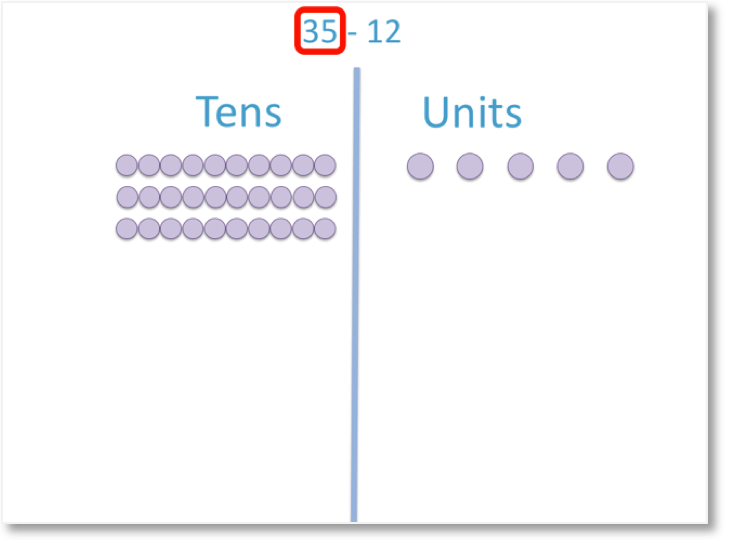We will subtract 12.

12 is made up of 2 individual units (ones) and 1 group of 10.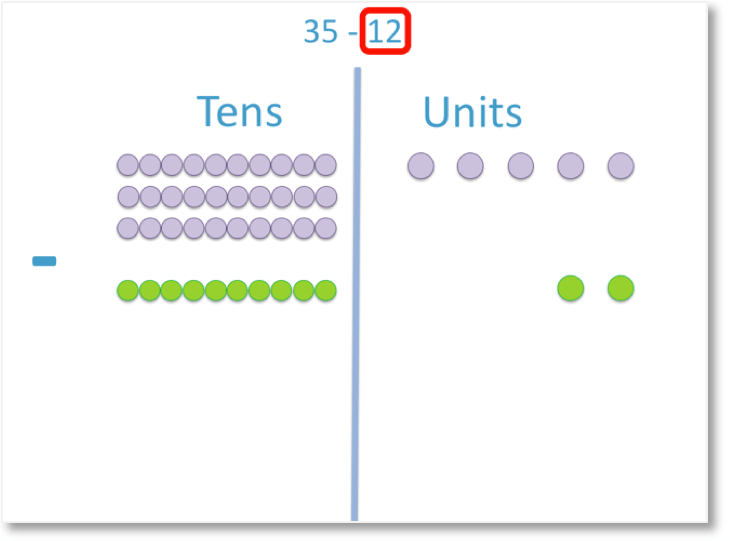We now subtract the twelve from the thirty-five.

To perform the subtraction, start in the units column and move to the left.

In the units column, 5 – 2 = 3.Now we have subtracted the digits in the units column, we move left to the tens column.

3 – 1 = 2Here is the overall process of subtraction.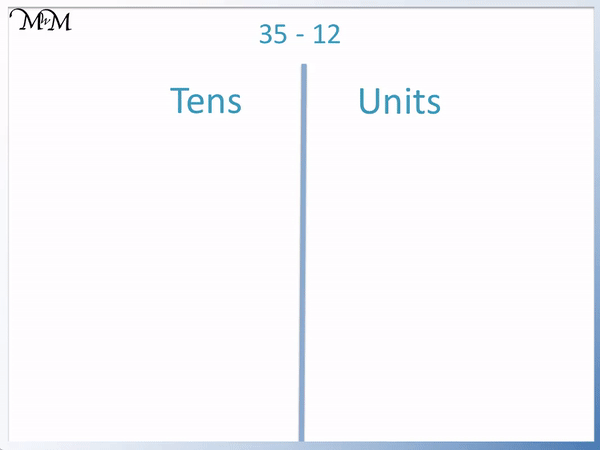35 – 12 = 23

We will now look at the same example but with the correct written method.

When teaching vertical column subtraction, it is recommended that you use paper with large boxes to write each digit in. Grid or graph paper is best. Using grid paper helps to keep the digits of each number in line when subtracting them.

We write the largest number first. 35 is larger than 12. We write each digit in a separate box.

We write the smallest number below. 12 is written directly below 35.

We write the units digit below the units digit of the other number, so 2 below 5.

We write the tens digit below the tens digit of the other number, so 1 below 3.

We draw to horizontal lines to write the answer between.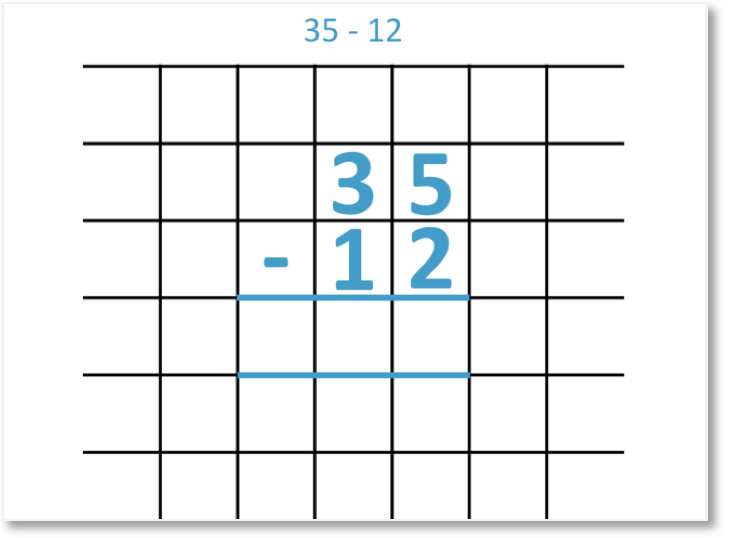We subtract the digits in the units column.

5 – 2 = 3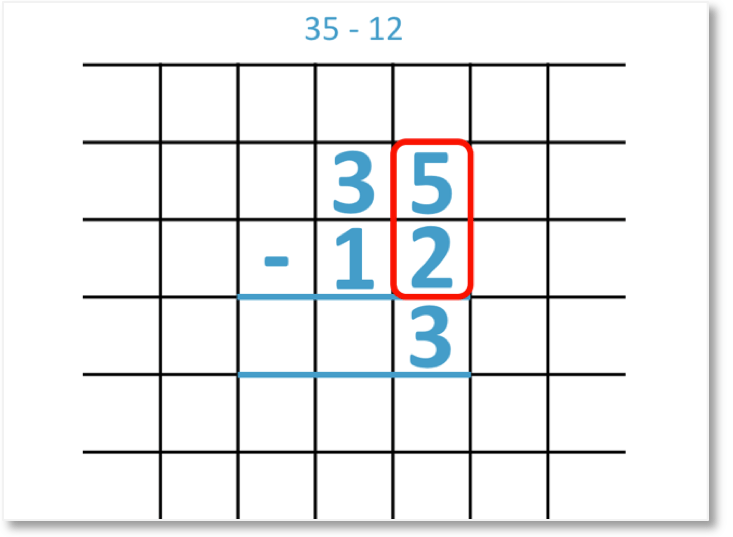Now we subtract the digits in the tens column.

3 – 1 = 2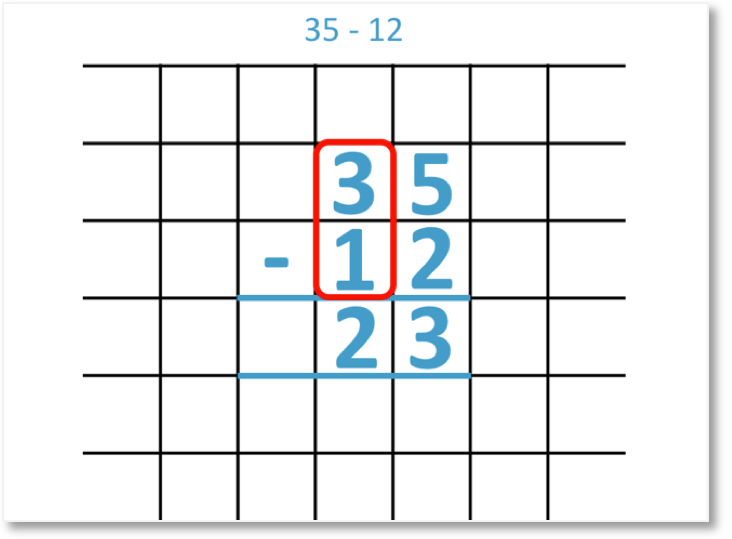Here is the full column subtraction process.We will look at another example of the column subtraction method.

Here we have 78 – 43.

We write each digit in a separate box, with the smaller number written below the larger number.Subtracting the digits in the units column, we have:

8 – 3 = 5

Subtracting the digits in the tens column, we have:

7 – 4 = 3

78 – 43 = 35

Here is another example of a subtraction without borrowing.

We have 64 – 34.

We line up the digits in their place value columns.

64 is larger than 34 and so, we write 64 above the 34.Starting in the units column, 4 – 4 = 0.

We need to write a zero in the answer box here.

Subtracting the digits in the tens, we have:

6 – 3 = 0

Therefore, 64 – 34 = 30.Now try our lesson on 2-Digit Column Subtraction with Borrowing / Regrouping where we learn how to use vertical column subtraction when regrouping is involved.